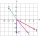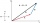# Angle between vectors

Find the angle between the given vectors to the nearest tenth of a degree. u = (-22, 11) and v = (16, 20)

Result

A =  0 °

#### Solution:

$u = \sqrt{ (-22)^{ 2 }+11^{ 2 } } = 11 \ \sqrt{ 5 } \doteq 24.5967 \ \\ v = \sqrt{ 16^{ 2 }+20^{ 2 } } = 4 \ \sqrt{ 41 } \doteq 25.6125 \ \\ s = (-22) \cdot \ (16)+(11) \cdot \ (20) = -132 \ \\ A = \frac{ 180^\circ }{ \pi } \cdot \arccos(s/(u \cdot \ v)) = \frac{ 180^\circ }{ \pi } \cdot \arccos((-132)/(24.5967 \cdot \ 25.6125)) \doteq 102.0948 = 0 ^\circ$

Leave us a comment of this math problem and its solution (i.e. if it is still somewhat unclear...):Be the first to comment!#### Following knowledge from mathematics are needed to solve this word math problem:

For Basic calculations in analytic geometry is helpful line slope calculator. From coordinates of two points in the plane it calculate slope, normal and parametric line equation(s), slope, directional angle, direction vector, the length of segment, intersections the coordinate axes etc. Two vectors given by its magnitudes and by included angle can be added by our vector sum calculator. Most natural application of trigonometry and trigonometric functions is a calculation of the triangles. Common and less common calculations of different types of triangles offers our triangle calculator. Word trigonometry comes from Greek and literally means triangle calculation. Pythagorean theorem is the base for the right triangle calculator.

## Next similar math problems:

1. Scalar dot productCalculate u.v if |u| = 5, |v| = 2 and when angle between the vectors u, v is: a) 60° b) 45° c) 120°
2. Vector sumThe magnitude of the vector u is 12 and the magnitude of the vector v is 8. Angle between vectors is 61°. What is the magnitude of the vector u + v?
3. Find the 10Find the value of t if 2tx+5y-6=0 and 5x-4y+8=0 are perpendicular, parallel, what angle does each of the lines make with the x-axis, find the angle between the lines?
4. CuboidsTwo separate cuboids with different orientation in space. Determine the angle between them, knowing the direction cosine matrix for each separate cuboid. u1=(0.62955056, 0.094432584, 0.77119944) u2=(0.14484653, 0.9208101, 0.36211633)A ship travels 84 km on a bearing of 17°, and then travels on a bearing of 107° for 135 km. Find the distance of the end of the trip from the starting point, to the nearest kilometer.
6. Reference angleFind the reference angle of each angle:
7. TriangleTriangle KLM is given by plane coordinates of vertices: K[11, -10] L[10, 12] M[1, 3]. Calculate its area and itsinterior angles.
8. Greatest angleCalculate the greatest triangle angle with sides 197, 208, 299.
9. Vector - basic operationsThere are given points A [-9; -2] B [2; 16] C [16; -2] and D [12; 18] a. Determine the coordinates of the vectors u=AB v=CD s=DB b. Calculate the sum of the vectors u + v c. Calculate difference of vectors u-v d. Determine the coordinates of the vector w.
10. Scalar productCalculate the scalar product of two vectors: (2.5) (-1, -4)Given that P = (5, 8) and Q = (6, 9), find the component form and magnitude of vector PQ.Given vector OA(12,16) and vector OB(4,1). Find vector AB and vector |A|.Determine coordinates of unit vector to vector AB if A[-6; 8], B[-18; 10].Determine coordinates of the vector u=CD if C[19;-7], D[-16,-5].Determine the coordinate of a vector u=CD if C(19;-7) and D(-16;-5)Line p passing through A[-10, 6] and has direction vector v=(3, 2). Is point B[7, 30] on the line p?We want to prove the sentence: If the natural number n is divisible by six, then n is divisible by three. From what assumption we started?